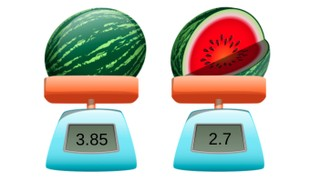Estimated and exact difference with decimal numbers with 1 and 2 decimal places

# Estimated and exact difference with decimal numbers with 1 and 2 decimal places8,000 schools use Gynzy92,000 teachers use Gynzy1,600,000 students use Gynzy

## General

Students learn to estimate the difference and to find the exact difference of subtraction problems with decimal numbers with 1 and 2 decimal places.

5.NBT.B.7

## Relevance

It is useful to be able to estimate the difference and find the exact difference of decimal numbers, so you can use the estimate to check the exact difference. You can use this to determine how much you have left after you take something away.

## Introduction

The interactive whiteboard shows sets of three decimal numbers. Students must determine which of the three is the smallest number. They can write A, B, or C. Remind students that numbers with two decimal places are not always larger than numbers with 1 decimal place.

## Development

To be able to estimate the difference with decimal numbers with 1 and 2 decimal places, students must be able to round decimal numbers to whole numbers. Explain that they can do this by looking on a number line to see which whole number a decimal number is closest to. You can also explain that with larger numbers it can be easier to round to a tens or hundreds number, to make the subtraction problem easier. Next explain how to subtract numbers with 1 and 2 decimal places. The first step is to make sure that both numbers have the same number of decimal places. You can do this by adding a 0 to the hundredths place. Show that you can subtract on the number line when you split the subtrahend and take it away from the first number in parts. Show students that they can also subtract without a number line, by splitting both numbers and subtracting the whole numbers, subtracting the numbers after the decimal point, and then adding those differences together. Give students a few problems including a story problem discuss how you can use both methods to find the difference. Encourage students to explain their strategy.

To check that students are able to estimate the difference and find the exact difference of decimal numbers with 1 and 2 decimal places, you can ask the following questions.
- How do you round a number if you want to estimate a difference?
- How do you subtract decimal numbers if you want to know the exact difference?
- What is useful to do as a first step when subtracting a number with 2 decimal places from a number with 1 decimal place?

## Guided practice

Students are given subtraction problems in which they are asked to estimate and then give the exact difference of decimal numbers with 1 and 2 decimal places. They are also given a story problem to estimate and find the exact difference of.

## Closing

Remind students that it is important to be able to estimate a difference, because you use it as a tool to check your exact difference. The two numbers should be close together. Check that students know how to estimate and find the exact difference between decimal numbers with 1 and 2 decimal places by asking them to solve a few problems and explain their strategy. Remind them to add a zero to the hundredths place to turn the number with 1 decimal place into a number with 2 decimal places. Next two story problems are given, and ask students to solve individually or in pairs. Have students explain how they round and what strategy they use.

## Teaching tips

Students who have difficulty with this learning goal can be supported by making use of a number line. They should first practice rounding decimal numbers to whole numbers, because then they build confidence that their estimated difference is a good check for their exact difference. You can also encourage students to take smaller jumps, or to split the subtrahend into smaller portions to take away from the minuend.

### The online teaching platform for interactive whiteboards and displays in schools

• Save time building lessons

• Manage the classroom more efficiently

• Increase student engagement